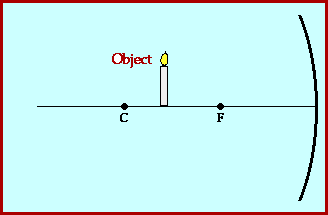## Ray Diagrams for Concave Mirrors - Case C

### Object Located Between the Center of Curvature and the Focal Point

Ray diagrams are useful tools for determining the location of an image as produced by a concave mirror. To determine the location of an image using a ray diagram, at least two sets of incident and reflected rays must be constructed for strategic positions on the object. The image of an object is the location where light rays from that object intersect upon reflecting from a mirror. By constructing at least two sets of incident and reflected rays, this image location can easily be found.

If the object is represented by an arrow, then it is common practice to pick the two extreme positions (the top and the bottom of the arrow) as starting points for the incident and reflected rays. Ray construction will result in the determination of the image locations for these two extreme positions on the object. The complete image is merely an arrow connecting these two image locations. This task is further simplified if the object is positioned as an arrow standing upon the principal axis of the mirror. If this is the case, then the image will be standing upon the principal axis of the mirror (and either inverted or upright).

Of all the rays which emanate from the top of the object arrow and are incident to the mirror, there are two rays whose behavior at the mirror surface can be easily predicted. These are the two incident rays which are used in the ray construction. One of the rays moves parallel to the principal axis and reflects through the focal point. The second ray passes through the focal point on the way to the mirror and reflects parallel to the principal axis. All concave and convex mirror ray diagrams can be constructed from knowledge of the behavior of these two rays.In the animation above, a right-side-up object is located above the principal axis between the center of curvature (C) and the focal point (F). The ray diagram shows that the image of this object is located as an upside-down image positioned beyond the center of curvature (C). In fact, it can be generalized that anytime the object is located between C and F, the image will be located beyond the center of curvature as well. In such cases, the image will be inverted and larger in size than the object. Such images are called real images because they are formed by the actual convergence of reflected light rays at the image location. Real images are always formed on the same side of the mirror as the object.

For more information on the ray nature of light, visit The Physics Classroom Tutorial. Detailed information is available there on the following topics:

Why is an Image Formed?

The Anatomy of a Curved Mirror

Reflection of Light and Image Formation

Two Rules of Reflection for Concave Mirrors

Ray Diagrams - Concave Mirrors

Image Characteristics for Concave Mirrors

The Mirror Equation - Concave Mirrors